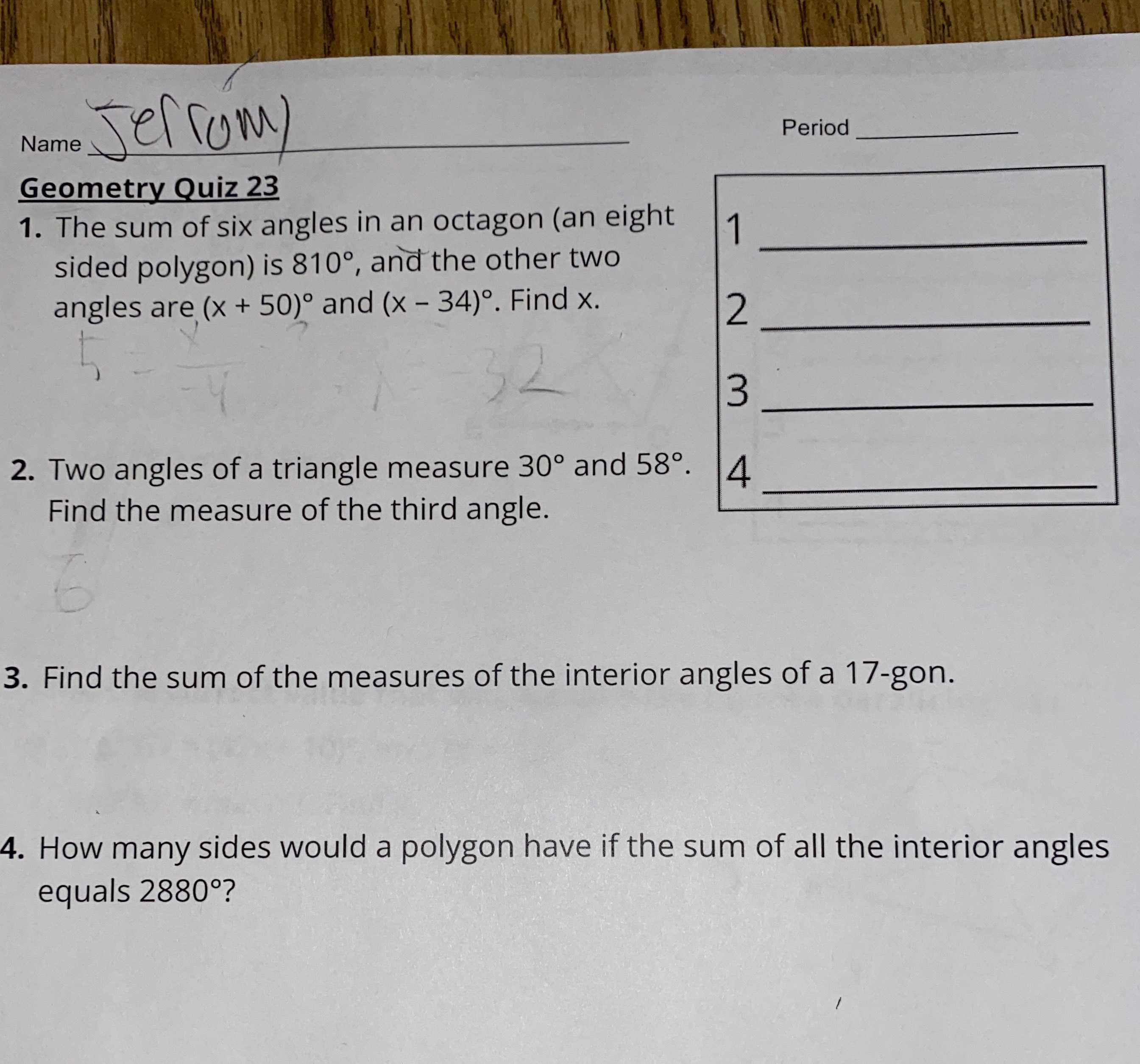### ¿Todavía tienes preguntas de matemáticas?

Pregunte a nuestros tutores expertos
Algebra
Pregunta1. The sum of six angles in an octagon (an eight sided polygon) is $$810 ^ { \circ }$$ , and the other two angles are $$( x + 50 ) ^ { \circ }$$ and $$( x - 34 ) ^ { \circ }$$ . Find $$x$$ . 2. Two angles of a triangle measure $$30 ^ { \circ }$$ and $$58 ^ { \circ }$$ . Find the measure of the third angle. 3. Find the sum of the measures of the interior angles of a 17-gon. equals $$2880 ^ { \circ }$$ ? 4. How many sides would a polygon have if the sum of all the interior angles equals $$2880 ^ { \circ }$$ ?

1)$$810° + (x+ 50)° + (x- 34)° = 1080°$$

$$x= 127°$$

2)third angle = $$180° - 30° - 58° = 92°$$

3)sum = $$(17- 2)180° = 2700°$$

4)$$2880° = (n- 2)180°$$

n=18 sides

Solución
View full explanation on CameraMath App.